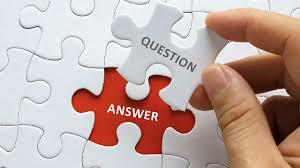# Teaching Pronunciation/Grammar/Vocabulary Quiz

7 QuestionsSettings.

Related Topics
• 1.
The change of tones in speaking is called
• A.

A. Morphology

• B.

B. Intonation

• C.

C. Synonym

• D.

d. Antonym

• 2.
Indicate two main methods of teaching grammar? (Check all that apply)
• A.

A. Inductive

• B.

B. Deductive

• C.

C. Explicit

• D.

D. Implicit

• 3.
What is this grammar method called when students are instructed to produce grammar rule?
• A.

A. Inductive

• B.

B. Deductive

• C.

C. Comprehensive

• D.

D. Exposure

• 4.
What is this grammar method called when students are firstly given grammar rule and asked to practice with it later?
• A.

A. Inductive

• B.

B. Deductive

• C.

C. Comprehensive

• D.

D. Exposure

• 5.
What is the strongest point of teaching grammar with inductive method?
• A.

A. Prolonged memory of the grammar point

• B.

B. Shortening time of explanation

• C.

C. No need to expose students to various examples.

• D.

D. When time for explaining grammar point is lenghthy enough

• 6.
Indicate two most proproriate casess that pronunication should be taught? (Check all that apply)
• A.

• B.

B. Teaching listening

• C.

C. Teaching vocabulary

• D.

D. When certain pronunciation is problematic to the learners

• 7.
What is this teaching method called in which teacher tries to raise students' interest before actually teaching students the learning point. Students, then, activate the lesson by producing based on what they have just taught.
• A.

A. ESA Patchwork

• B.

B. Boomerang

• C.

C. ESA straigh arrows sequence